Select Page

# CBSE MCQ in English Answers for Maths 12 Science Three Dimensional Geometry

CBSE MCQ in English Answers for Maths 12 Science Three Dimensional Geometry to enable students to get Answers in a narrative video format for the specific question.

Expert Teacher provides CBSE MCQ Answers for Maths 12 Science Three Dimensional Geometry through Video Answers in English language. This video solution will be useful for students to understand how to write an answer in exam in order to score more marks. This teacher uses a narrative style for a question from Three Dimensional Geometry not only to explain the proper method of answering question, but deriving right answer too.

Please find the question below and view the Answer in a narrative video format.

Question:

## Similar Questions from CBSE, 12th Science, Maths, Three Dimensional Geometry

Question 1 : Write the sum of intercepts cut off by the planeon the three axes.  (View Answer Video)

Question 3 : Find the angle between the planes 7x + 2y + 6z = 15 and 3x - y + 10z = 17. (View Answer Video)

Question 4 : Find the vector equation of the plane with intercepts 3, -4 and 2 on x, y and z-axis respectively. (View Answer Video)

Question 5 : Find the equation of the plane that contains the point (1, -1, 2) and is perpendicular to both the planes 2x + 3y - 2z = 5 and x + 2y - 3z = 8. Hence find the distance of point P(-2, 5, 5) from the plane obtained above. (View Answer Video)

### Differential Equations

Question 1 : Find the sum of the order and the degree of the following differential equation:(View Answer Video)

Question 2 : Find the particular solution of the differential equationgiven that y = 1 when x = 0.  (View Answer Video)

Question 3 : Find the general solution of differential equation(View Answer Video)

Question 4 : Solve the differential(View Answer Video)

Question 5 : Solve the differential equation(View Answer Video)

### Probability

Question 1 :  A and B throw a pair of dice alternatively, till one of them gets a total of 10 and wins the game. Find their respective probabilities of winning, if A starts first.   (View Answer Video)

Question 2 : If A and B are two events such that P(A)=0.3, P(B)=0.6 and P(B/A)=0.5, find P(A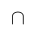B). (View Answer Video)

Question 3 : If E and F are two events such that,, Find P(not E and not F). (View Answer Video)

Question 4 : A die is thrown 6 times. If 'getting an odd number' is a 'success', what is the probability of obtaining:
atleast 5 successes?

Question 5 : If P(A)=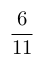, P(B)=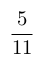and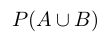=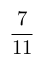, find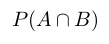. (View Answer Video)

### Relations and Functions

Question 1 : Letdefined as f(x) = x be an identity function. Then, (View Answer Video)

Question 2 : Number of binary operations on the set { a, b} are: (View Answer Video)

Question 3 : Let * be the binary operation on N given by a * b = LCM of a and b. Find 5 * 7. (View Answer Video)

Question 4 : Let * be the binary operation on N given by a * b = LCM of a and b. Find 5 * 7. (View Answer Video)

Question 5 : Let * be the binary operation on N given by a * b = LCM of a and b. Find 20 * 16. (View Answer Video)